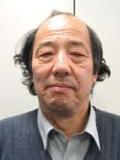# Akihiro TsuchiyaAkihiro Tsuchiya

I started my mathematical research in the latter half of the 1960s in the field of differential topology. The research of differential topology was in the final phase of the development. I succeeded to determine completely the characteristic classes of spherical fiber spaces, which was a main problem in this field. In the latter half of the 1980s I began to study the mathematical foundations of conformal field theory. Conformal field theory is a two dimensional quantum field theory that describes the critical phenomena in two dimensional statistical physics. It was found by three Russian physicists in the beginning of 1980s, and the close relationship with string theory in elementary particle physics was clarified afterwards. I developed conformal field theory by using methods and techniques of modern mathematics such as representation theory of infinite dimensional algebra, the theory of D-modules and the theory of moduli spaces in algebraic geometry. Through these results I gave new insights and directions to modern mathematics.